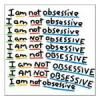# Search the Community

Showing results for tags 'use'.

• ### Search By Tags

Type tags separated by commas.

### Forums

• News & Announcements
• News
• Project Zomboid
• General Discussions
• Bug Reports
• PZ Support
• PZ Multiplayer
• PZ Community & Creativity
• PZ Suggestions
• PZ Modding
• Tutorials & Resources
• Mods
• Items
• Mapping
• Mod Ideas and Requests
• General Games Development
• Indie Scene
• Other Discussions
• General Discussion
• Forum Games & Activities

• 0 Replies

• 0 Views

### Interests

Found 3 results

1.## items Sock to Ripped Sheets and Crayons as a writing tool

There could be added a small use for those two items - first it always got me curious why can I rip sheet underwear, but not socks... It should be usable just like all the other clothing items for that small use (it shouldn't make any major difference gameplay-wise, seeing how rare socks are and the cloths for rip sheets are widely available on zombies in the first place, but it would give this item some use, who knows, maybe saving someone's wound in a pinch . The second thing is crayons, which I think can not be used as a writing tool (correct me if I'm wrong, I don't have a journal to check on my save game). Obviously, they wouldn't make any kind of improvised weapon like pen and pencil, but they certainly could serve as a writing tool for those who feel the need to write some journals - and also giving a use to yet another item :). Both the requests should be easy enough to implement too, as there is no need to add any new graphic, model or recipe - just add them as another item that can do things already implemented.
2.## Drainable Item UseDelta chart (make-shift chart)

The use delta is the amount of uses that Drainable items have. The formula is 1 divided by delta number = Number of uses. Thank you 7Roses . I will make a complete and better chart at some point. Unless someone else wants to use a calculator or their head to make it for us. 1=1 .1 = 9 .2 = 5 .3 = .4 = .5 = .6 = .7 = .8 = .9 =.01 = .02 = ALOT .03 = 33 .04 = 24 .05 = 19 .06 = 16 .07 = 14 .08 = 12 .09 = 11 .10 = .11 = .12 = .13 = .14 = .15 = 6 .16 = .17 = .18 = .19 = .20 = 5 .21 = .22 = .23 = .24 = .25 = 4 .26 = .27 = .28 = .29 = .30 = .31 = .32 = .33 = .34 = .35 = .36 = .37 = .38 = .39 = .40 = .41 = .42 = .43 = .44 = .45 = .46 = .47 = .48 = .49 = .50 = .51 = .52 = .53 = .54 = .55 = .56 = .57 = .58 = .59 = .60 = .001 = .002 = .003 = .004 = .005 = .006 = .007 = .008 = .009 = .010 = .011 = .012 = .013 = .014 = .015 = .016 = .017 = .018 = .019 = .020 = .021 = .022 = .023 = .024 = .025 = .026 = .027 = .028 = .029 = .030 = .031 = .032 = .033 = .034 = .035 = .036 = .037 = .038 = .039 = .040 = .041 = .042 = .043 = .044 = .045 = .046 = .047 = .048 = .049 = .050 = .051 = .052 = .053 = .054 = .055 = .056 = .057 = .058 = .059 = .060 =
3. So... Umbrellas, not rare but not super-common as loot in houses and the stores. It would require both hands to use and would open in three seconds but take ten seconds to shake dry and close. Both of those timers would require you to be standing still. The noise from the water hitting the umbrella would make more noise than the rain around it making you a little more noticeable when walking around in the rain if zombies are about. It would be usable as a very limited bat like weapon and would break pretty fast, maybe one to three uses. It would have the close combat jaw kill move like knives, maybe four to five uses. If in multi-player, two or three people can stand under it and not get wet. It would wear out over time from the wear and tear and from the water damaging it (long-term). And if hot and sunny, you stay cool when using it.
×
×
• Create New...